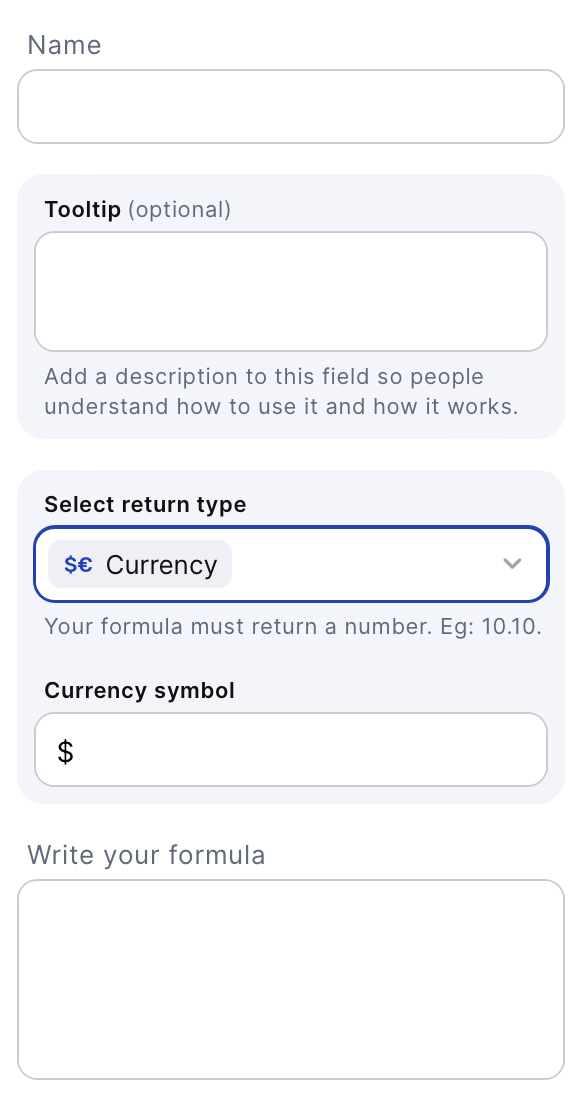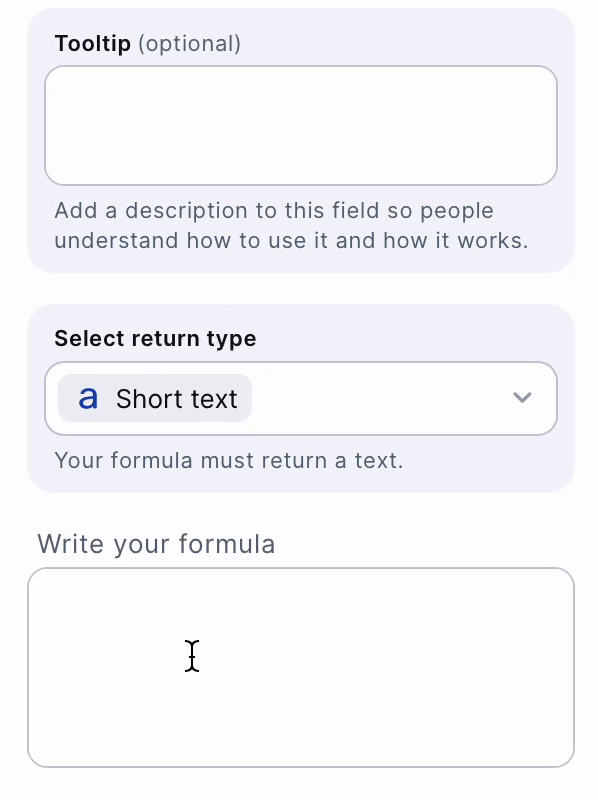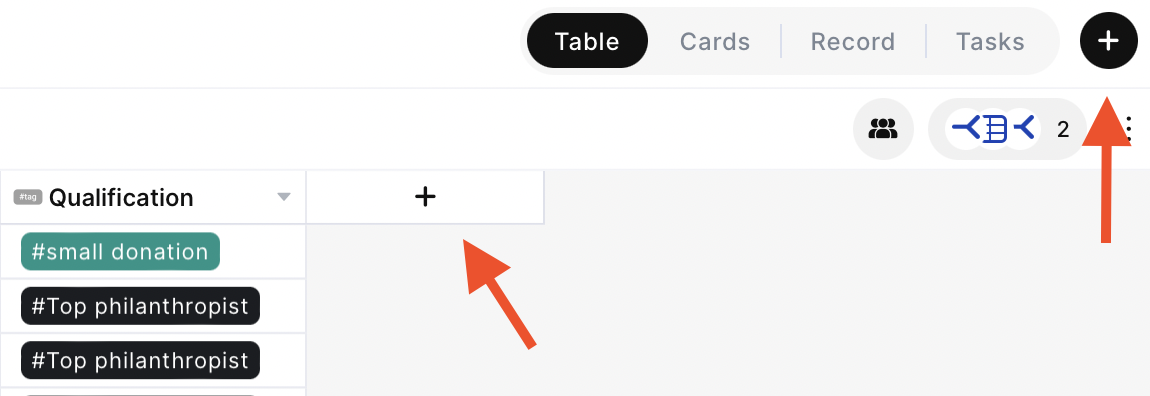# Formula

Free form formula field to create your own formula.# What is the Formula field?

The Formula Field supports both basic operators and complex functions. You can use fixed numbers, fields of the record itself and even fields from connected records to build your formula!# FunctionsFunctions are predetermined complex operations that will return you a value. The ones currently supported are explained below:

IF: returns a value if a condition is true and a different value if it's false.

For more details and examples: https://www.w3schools.com/mysql/func_mysql_if.asp

``````IF(condition, value_if_true, value_if_false) ;
``````

CONCAT: allows you to concatenate text, like combining pine and apple to return pineapple.

For more details and examples: https://www.w3schools.com/mysql/func_mysql_concat.asp

``````CONCAT(expression1, expression2, expression3,...);
``````

ROUND: rounds a number to a given number of decimal places.

For more details and examples: https://www.w3schools.com/mysql/func_mysql_round.asp

``````ROUND(number, decimals);
``````

POW function returns the value of a number raised to the power of another number.

For more details and examples: https://www.w3schools.com/mysql/func_mysql_pow.asp

``````POW(x, y);
``````

NOW() function returns the current date and time.

For more details and examples: https://www.w3schools.com/mysql/func_mysql_now.asp

``````NOW();
``````

DATE_FORMAT function formats a date as specified.

For more details and examples: https://www.w3schools.com/mysql/func_mysql_date_format.asp

``````DATE_FORMAT(date, format);
``````

TIME_FORMAT function formats a time by a specified format.

For more details and examples: https://www.w3schools.com/mysql/func_mysql_time_format.asp

``````TIME_FORMAT(time, format);
``````

DATE_ADD function adds a time/date interval to a date and then returns the date.

For more details and examples: https://www.w3schools.com/mysql/func_mysql_date_add.asp

``````DATE_ADD(date, INTERVAL value addunit);
``````

DATE_SUB function subtracts a time/date interval from a date and then returns the date.

For more details and examples: https://www.w3schools.com/mysql/func_mysql_date_sub.asp

``````DATE_SUB(date, INTERVAL value interval);
``````

DATEDIFF function returns the number of days between two date values.

For more details and examples: https://www.w3schools.com/mysql/func_mysql_datediff.asp

``````DATEDIFF(date1, date2);
``````

MICROSECOND function returns the microsecond part of a time/datetime (from 0 to 999999).

For more details and examples: https://www.w3schools.com/mysql/func_mysql_microsecond.asp

``````MICROSECOND(datetime);
``````

SECOND function returns the seconds part of a time/datetime (from 0 to 59).

For more details and examples: https://www.w3schools.com/mysql/func_mysql_second.asp

``````SECOND(datetime);
``````

MINUTE function returns the minute part of a time/datetime (from 0 to 59).

For more details and examples: https://www.w3schools.com/mysql/func_mysql_minute.asp

``````MINUTE(time);
``````

HOUR function returns the hour part for a given date (from 0 to 838).

For more details and examples: https://www.w3schools.com/mysql/func_mysql_hour.asp

``````HOUR(time);
``````

DAY function returns the day of the month for a given date (a number from 1 to 31).

For more details and examples: https://www.w3schools.com/mysql/func_mysql_day.asp

``````DAY(date);
``````

MONTH function returns the month part for a given date (a number from 1 to 12).

For more details and examples: https://www.w3schools.com/mysql/func_mysql_month.asp

``````MONTH(date);
``````

YEAR function returns the year part for a given date (a number from 1000 to 9999).

For more details and examples: https://www.w3schools.com/mysql/func_mysql_year.asp

``````YEAR(date);
``````

DATE function extracts the date part from a datetime expression.

For more details and examples: https://www.w3schools.com/mysql/func_mysql_date.asp

``````DATE(expression);
``````

### 🚧Parentheses

You can use parenthesis to define the order of operations, for example: ( {{pre_total_2}}*{{amount}} ) - {{discount_2}}

# Basic operators

Addition, Subtraction, Division and Multiplication. classic operators you can use along with functions.

Subtraction: -
Division: /
Multiplication: *

# How to create a Formula field

To create a Formula field:

• On table mode, click on the last table header with a "+", press (F) or click on the + on the upper right and this will open the field creation page• Search for the "Formula" option and click on it
• Choose a name for your field and write the desired formula

### 📘

You can delete or edit by clicking on the table header name and choosing one of the options

# Common use cases

The Formula field is very flexible and allows you to choose the return type of the formula. It can be used in a lot of different situations:

For example:

• In a “Leads” table, the formula field allows you to combine the name of the contact, the name of the contact’s company and a message text in a formula field to help send an automated email message.

• In a “Leads” table, the formula field allows you to get the year of a date field that was used to save the time that the lead was contacted and help create a new filter for the leads.

• In a “Inventory” table, it can be used to calculate the difference between the quantity of products in the inventory and the lowest quantity required to be in the inventory. This helps visualize the quantity of products that can be sold.

• In a “Financial Statement” table, it can be used to calculate the difference between the value of a record and taxes and have faster the net value.

What’s Next